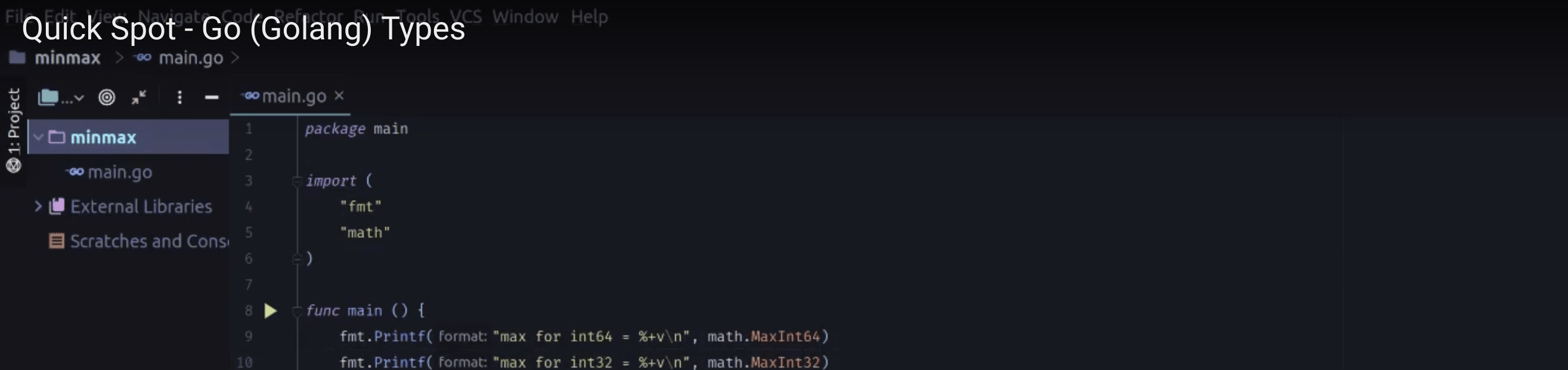# A Short Spot on Coding up Go Types Sizing LimitsIn an effort, finally, to get around to putting more content together I’ve created this video. It’s the first of many that I’ll put together. This one is a quick coding example of getting the maximum size of particular types in Go. At just about a minute, it’s a quick way to pick up a way to do something coding. If you like this video, do leave a comment, if you thought it wasn’t useful leave a comment. Either way I’d like to read the over under on material like this and if it is or isn’t useful (or entertaining) for you. It’ll mostly be just little tips n’ tricks on how to get things done.

The code gist.

This file contains bidirectional Unicode text that may be interpreted or compiled differently than what appears below. To review, open the file in an editor that reveals hidden Unicode characters.

 package main import ( "fmt" "math" ) const( MinUint uint = 0 // all zeroes // Perform a bitwise NOT to change every bit from 0 to 1. MaxUint = ^MinUint // all ones // Shift the binary number to the right to get the high bit to zero MaxInt = int(MaxUint >> 1) // all ones except high bit MinInt = ^MaxInt // all zeroes except high bit. ) func main() { // integer max fmt.Printf("max for int64 = %+v\n", math.MaxInt64) fmt.Printf("max for int32 = %+v\n", math.MaxInt32) fmt.Printf("max for int16 = %+v\n", math.MaxInt16) fmt.Printf("max for int8 = %+v\n", math.MaxInt8) // integer min fmt.Printf("min for int64 = %+v\n", math.MinInt64) fmt.Printf("min for int32 = %+v\n", math.MinInt32) fmt.Printf("min for int16 = %+v\n", math.MinInt16) fmt.Printf("min for int8 = %+v\n", math.MinInt8) // Added parts that aren't in the video! fmt.Printf("max float64 = %+v\n", math.MaxFloat64) fmt.Printf("max float32 = %+v\n", math.MaxFloat32) fmt.Printf("smallest nonzero float64 = %+v\n", math.SmallestNonzeroFloat64) fmt.Printf("smallest nonzero float32 = %+v\n", math.SmallestNonzeroFloat32) fmt.Printf("max uint = %+v\n", MaxUint) fmt.Printf("min uint = %+v\n", MinUint) fmt.Printf("max int = %+v\n", MaxInt) fmt.Printf("min int = %+v\n", MinInt) }

view raw

main.go

hosted with ❤ by GitHub